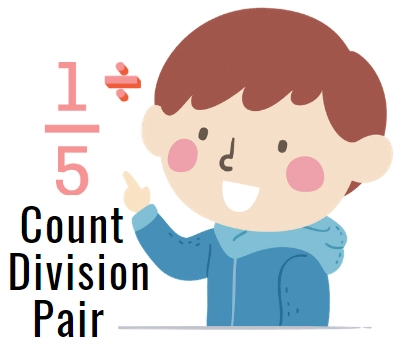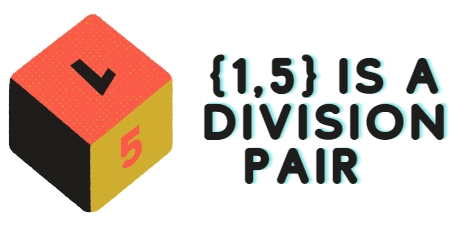New update is available. Click here to update.
Last Updated: Jul 1, 2023

# Count Divisible Pairs in an ArrayAyushi Goyal0 upvotes

## Introduction

In this article, we will discuss the problem to count divisible pairs in an array and briefly discuss the approach to be used and its time and space complexity. Before discussing count divisible pairs in an array problem, let’s first discuss what an array means.

Array: It is a linear data structure consisting of a collection of values called elements of an array, stored in a contiguous memory location, and can be accessed using array indexes.In this problem, we are given an array of any size(provided by the user), and our task is to count division pairs in an array. You may be wondering🤔 what this division pair is. Here is the answer 😃👉

Division pair: If one element of a pair divides another element, then such a pair is called a division pair.

Example:Recommended topic, kth largest element in an array and  Euclid GCD Algorithm

## Native Approach

Using brute force, iterating through each element of an array and checking if pair (‘i’,’j’) are such that ‘a[i]’ % ‘a[j]’ = 0 or not.

### Algorithm

• Take array size as input ‘n’.
• Create an array of size ‘n’, lets say, ‘a[n]’.
• Take a temporary variable ‘count’ for counting division pairs.
• Start a for loop from ‘i’ = 0 to ‘n-1’.
• Inside this loop, start another for loop from ‘j’ = ‘i+1’ to ‘n-1’.
• Inside these loop check if ‘a[i]’ % ‘a[j]’ = 0 or ‘a[j]’ % ‘a[i]’ = 0.
• If the condition is true, increment the ‘count’ variable.
• Else continue.
• Return ‘count’.
• Print the result.

### Code in C++ 💻

``````/*
Program to count divisible pairs in an array.
*/

#include <iostream>
using namespace std;

int countPairs(int a[], int n)
{
int count = 0;

// Iterate through all pairs
for (int i=0; i<n; i++)
{
for (int j=i+1; j<n; j++)
{
// Increment count if one divides other
if (a[i] % a[j] == 0 ||  a[j] % a[i] == 0)
count++;
}

}
return count;
}

int main()
{
int n;
cout<<"Enter array size : ";
cin>>n;

int a[n];
cout<<"\nEnter array elements : ";
for(int i=0;i<n;i++)
cin>>a[i];
cout << countPairs(a, n);
return 0;
}``````

### Code in Java 💻

``````/*
Java program to count divisible pairs in an array.
*/

import java.util.Scanner;

class CodingNinjas {
static int countPair(int arr[],int n)
{
int count = 0;

// Iterate through all pairs
for (int i=0; i<n; i++)
{
for (int j=i+1; j<n; j++)
{
// Increment count if one divides other
if (a[i] % a[j] == 0 ||  a[j] % a[i] == 0)
count++;
}

}
return count;
}

// Driver Code
public static void main(String[] args)
{
Scanner sc=new Scanner(System.in);
System.out.print("Enter array size : ");
n=sc.nextInt();

int a[] = new int[n]
System.out.println("Enter array elements : ");
for(int i=0; i<n; i++)
{
a[i] = sc.nextInt();
}
System.out.print(countPairs(a, n));
}
} ``````

### Code in Python 💻

``````# Python3 program to count divisible pairs in an array.

def countPair(a, n) :
count = 0

# Iterate through all pairs
for i in range(0, n) :
for j in range(i+1, n) :
# Increment count if one divides other
if (a[i] % a[j] == 0 or a[j] % a[i] == 0) :
count+=1

return count

# Driver code
if __name__=='__main__':
n=int(input("Enter array size : "))
a=list(map(int, input("Enter array elements : ").strip().split()))
print(countPair(a, n))``````

### Time and Space Complexity

We have used two loops in the program, one is a single for loop iterating from 0 to ‘N’, This will take O(N) time complexity.

Another loop is a nested loop used to count division pairs in an array, this loop iterates from 0 to ‘N’ and ‘i + 1’ to ‘N’, this will take O(N*N) time complexity.

🎀Total time complexity = O(N) + O(N*N) = O(N*N)

🎀Space Complexity = O(N), for an array of size N.

Although the program is simple, but it is not an efficient solution for this problem, let’s discuss an efficient approach, that is by hashing.😉

## Efficient Approach

The approach is to count the total factors of all the elements in the array using an unordered map. Let’s first discuss the algorithm, and then we will move to code. 👇

### Algorithm

• Take array size as input ‘n’.
• Create an array of size ‘n’, let’s say, ‘a[n]’.
• Take a temporary variable ‘count’ and an unordered map ‘m’.
• Run a for loop from ‘i’ = 0 to ‘N-1’ and store the frequency of elements in the map. To access any element in 0(1) time complexity.
• Run another nested for loop from ‘i’ = 0 to ‘N-1’ and j = 1  to square root ‘a[i]’ to find all factor of a[i].
• Inside this loop check is ‘a[i]’%’j’ = 0, If this condition is true, then-
• Check if ‘a[i]] = ‘j’ x ‘j’.
• If this condition is also true then increment ‘count’ by ‘m[j]’.
• Otherwise increment ‘count’ by ‘m[j]’ + ‘m[a[i]/j]’.
• Otherwise, decrement ‘count’ by 1.
• Return the value of the ‘count’ variable.
• Print the result.

### Code in C++ 💻

``````/*
C++ code to count divisible pairs in an array
*/

#include <bits/stdc++.h>
using namespace std;
int countPair(int a[], int n)
{
int count = 0;

// Declaring an unordered_map
unordered_map<int, int>m;
for(int i=0;i<n;i++)
{
m[a[i]]++;
}

// Loop for finding all the divisors of each element
for(int i=0;i<n;i++)
{
for (int j=1;j<=sqrt(a[i]);j++)
{
if(a[i]%j==0)
{
if(arr[i]==j*j)
{
// If divisors are equal,then take only one
count+=freq[j];
}
else
{
// Else take both j and arr[i]/j
count+=freq[j]+ freq[arr[i]/j];
}
}
}

// Decrement by 1 as all the elements is divisible by itself
count--;
}
return count;
}

int main()
{
int n;
cout<<"Enter array size : ";
cin>>n;

int a[n];
cout<<"Enter array elements : ";
for(int i=0;i<n;i++)
{
cin>>a[i];
}
cout<<countPairs(a,n);
return 0;
}``````

### Code in Java 💻

``````/*
Java program to count divisible pairs in an array.
*/

import java.util.*;
import java.lang.Math;
public class Main{
static int countPair(int a[],int n)
{
int count=0;

Map<Integer, Integer>f = new HashMap<Integer, Integer>(10, 0.5f);
for(int i=0;i<n;i++)
{
if(f.containsKey(a[i]))
f.put(a[i], f.get(a[i]) + 1);
else
f.put(a[i],1);
}

for(int i=0;i<n;i++)
{
for (int j=1;j<=Math.sqrt(a[i]);j++)
{
if(a[i]%j==0)
{
if(a[i]==j*j)
count += f.get(a[i]);
else
count+=f.get(j)+ f.get(a[i]/j);
}
}
count--;
}
return count;
}

public static void main(String[] args)
{
Scanner sc=new Scanner(System.in);
System.out.print("Enter array size : ");
int n=sc.nextInt();

int a[] = new int[n];
System.out.println("Enter array elements : ");
for(int i=0; i<n; i++)
{
a[i] = sc.nextInt();
}
System.out.print(countPair(a, n));
}
}
``````

### Code in Python 💻

``````# Python3 program to count divisible pairs in an array.
import math
def countPair(a, n) :
m={}
for i in a:
m[i] = m.get(i, 0) + 1
count = 0
# Iterate through all pairs
for i in range(0, n) :
for j in range(1,(int)(math.sqrt(a[i])+1)) :
# Increment count if one divides other
if (a[i] % j == 0):
if(a[i] == j*j):
count += m.get(a[i])
else:
count +=m.get(j) + m.get(a[i]/j);
return count

# Driver code
if __name__=='__main__':
n=int(input("Enter array size : "))
a=list(map(int, input("Enter array elements : ").strip().split()))
print(countPair(a, n))
``````

### Time and space complexity

We have used three loops in the program. Two are single for loops iterating from 0 to ‘N’. This will take O(N) time complexity.

The third loop is a nested loop used to count factors of each element of the array, this loop iterates from 0 to ‘N’ and ‘1’ to the square root of ‘a[i]’. This will take O(N*√N) time complexity.

🎀Total time complexity = O(N) + O(N*√N) = O(N*√N)

🎀 Space complexity = O(N), for an array of size N.

Check out this problem - Pair Sum In Array.

### What is an array?

An array is a collection of similar values stored at contiguous memory locations. It is like a staircase, in which each value of placed at every step, and elements can be accessed by knowing the stair number (or index number).

### How many divisible pairs are present in array = {2,4,6,8}, mention each of them?

The given array has 4 divisible pairs, there are - {2,4}, {2,6}, {2,8}, {4,8}

### What is Hashing?

Hashing is a technique using which a given value can be transformed into another value. It makes searching more accessible and quicker.

### How can we calculate the frequency of elements in an array using a map?

For calculating the frequency, we need to iterate through every element of the array and add them to the map incrementing their previous values. Initially, a map is empty with all values assigned to zero. The code for the same is given below.

``````int a[] = {1,2,4,5,1,2,4}
unordered_map<int, int>m;
for(int i=0;i<n;i++)
{
m[a[i]]++;
}``````

## Conclusion

In this article, we see the implementation of the code to count divisible pairs in an array in different languages. We had also seen the example, time and space complexity, and output of the program on user input, along with an efficient approach to solving using hashing.